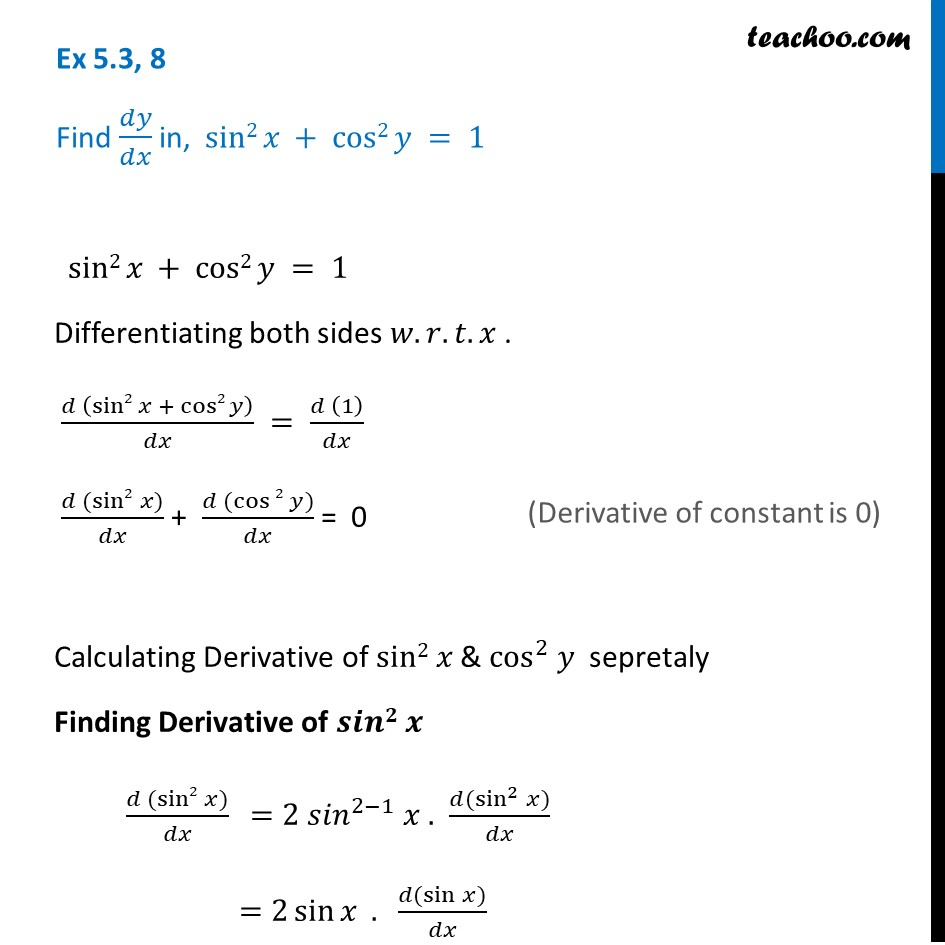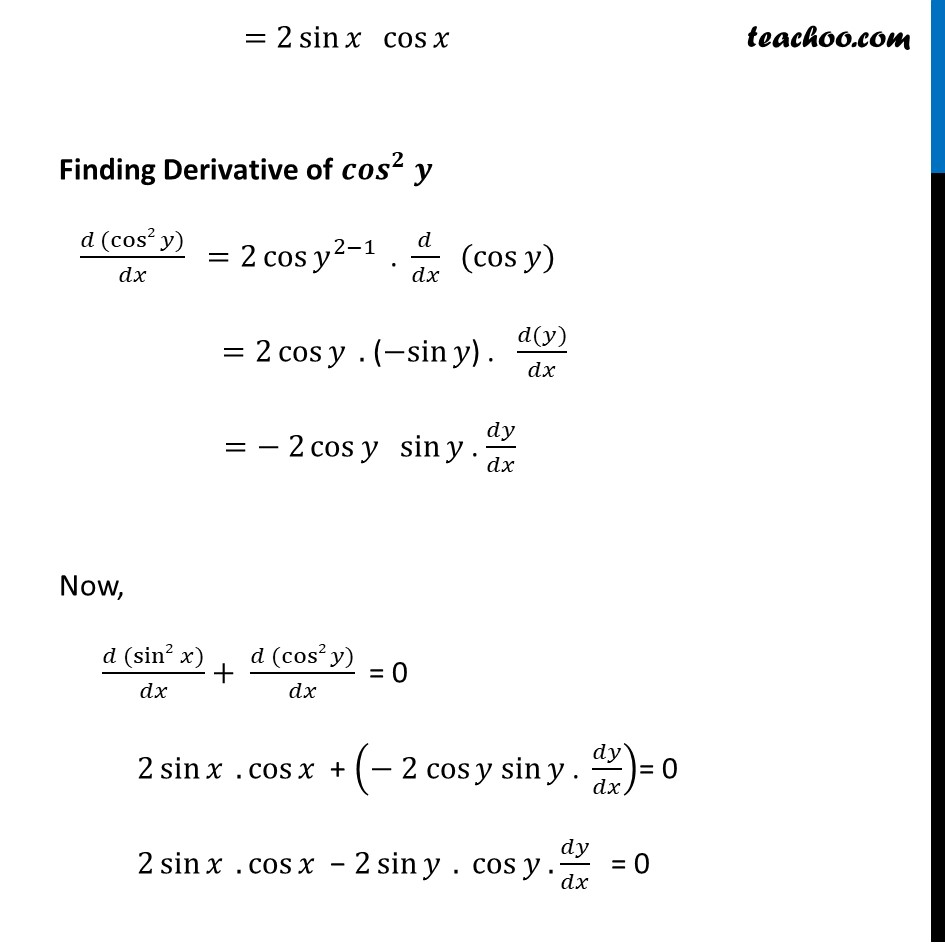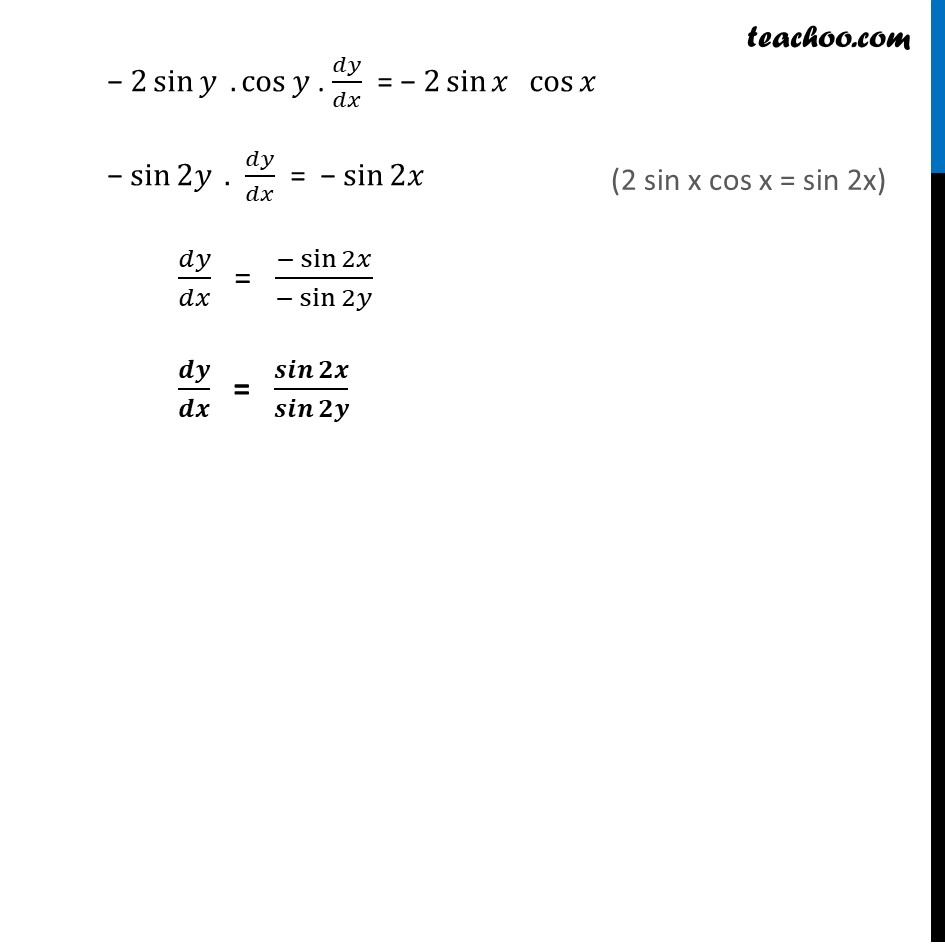Finding derivative of Implicit functions

Chapter 5 Class 12 Continuity and Differentiability
Concept wiseLearn in your speed, with individual attention - Teachoo Maths 1-on-1 Class

### Transcript

Ex 5.3, 8 Find 𝑑𝑦/𝑑𝑥 in, sin2 𝑥 + cos2 𝑦 = 1 sin2 𝑥 + cos2 𝑦 = 1 Differentiating both sides 𝑤.𝑟.𝑡.𝑥 . (𝑑 (sin2 𝑥 + cos2 𝑦))/𝑑𝑥 = (𝑑 (1))/𝑑𝑥 (𝑑 (sin2 𝑥))/𝑑𝑥 + (𝑑 (cos⁡2 𝑦))/𝑑𝑥 = 0 Calculating Derivative of sin2 𝑥 & cos^2 𝑦 sepretaly Finding Derivative of 𝒔𝒊𝒏𝟐 𝒙 (𝑑 (sin2 𝑥))/𝑑𝑥 =2〖 𝑠𝑖𝑛〗^(2−1) 𝑥 . (𝑑(sin^2⁡𝑥))/𝑑𝑥 =2 sin⁡𝑥 . (𝑑(sin⁡𝑥))/𝑑𝑥 (Derivative of constant is 0) =2 sin⁡〖𝑥 〖 cos〗⁡𝑥 〗 Finding Derivative of 〖𝒄𝒐𝒔〗^𝟐 𝒚 (𝑑 (cos2 𝑦))/𝑑𝑥 =2〖cos⁡𝑦〗^(2−1) ". " 𝑑/𝑑𝑥 " "(cos⁡𝑦) =2 cos⁡𝑦 . (−sin⁡𝑦) . (𝑑(𝑦))/𝑑𝑥 =− 2 cos⁡𝑦 sin⁡𝑦 . 𝑑𝑦/𝑑𝑥 Now, (𝑑 (sin2 𝑥))/𝑑𝑥+ (𝑑 (cos2 𝑦))/𝑑𝑥 = 0 2 sin⁡𝑥 .cos⁡𝑥 + (− 2 cos⁡𝑦 sin⁡𝑦 ". " 𝑑𝑦/𝑑𝑥)= 0 2 sin⁡𝑥 .cos⁡𝑥 − 2 sin⁡𝑦⁡〖 .〗 cos⁡𝑦 . 𝑑𝑦/𝑑𝑥 = 0 − 2 sin⁡𝑦⁡〖 .〗 cos⁡𝑦 . 𝑑𝑦/𝑑𝑥 = − 2 sin⁡𝑥 cos⁡𝑥 − sin⁡2𝑦⁡〖 .〗 𝑑𝑦/𝑑𝑥 = − sin⁡2𝑥 𝑑𝑦/𝑑𝑥 = 〖− sin〗⁡2𝑥/(−sin⁡2𝑦 ) 𝒅𝒚/𝒅𝒙 = 𝒔𝒊𝒏⁡𝟐𝒙/𝒔𝒊𝒏⁡𝟐𝒚 (2 sin x cos x = sin 2x)